Mathematics

# Horizontal and Vertical Line Test

4k views

## Table of Contents

 1 Introduction 2 Horizontal Line Test 3 Vertical Line Test 4 Horizontal Line Test, Vertical Line Test, One to One Function Test 5 Summary 6 FAQs

08 October 2020

Read time: 6 minutes

## Introduction

### What is the horizontal line test?

The horizontal line test is used to determine whether a function is injective.

When the horizontal line passes through a graph more than once, the function has more than one x value for at least one y value, so it cannot be one-to-one.

### What is the Vertical Line Test?

The vertical line test is a visual way to determine if a curve is a graph of a function or not.

Now let us explore both the horizontal line test and vertical line test in the blog.

## Horizontal line test

In geometry, a line is said to be horizontal if two points on the line have the same Y- coordinate points. It comes from the term “horizon”. It means that the horizontal lines are always parallel to the horizon.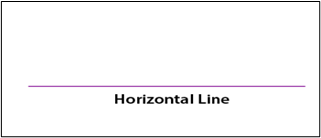The horizontal line test is the simplest method using which we can determine whether a given function has an inverse, but more importantly to find out if the inverse is also a function.

Remember that it is very possible that a function may have an inverse but at the same time, the inverse is not a function because it doesn’t pass the vertical line test.

The horizontal line intersects the graph of a function in all places at exactly one point, then the given function should have an inverse that is also a function. We can say that this function passes the horizontal line test.

The horizontal line test includes drawing a straight line that is mapped from left to right and it is parallel to the X-axis in the plane coordinate system. In other words, the horizontal line test is done using a straight line that does not make any intercept on the X-axis and it can have an intercept on the Y-axis is called the horizontal line.

### Horizontal Line Equation

When using the horizontal line test, it is important t know about the line used. The equation always takes the form:

y = k, where k is the y-intercept.

It is noted that the horizontal lines have the same slope, so the slope of the horizontal line is always zero.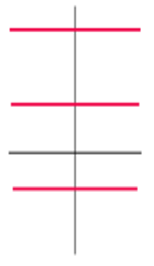### Horizontal Line Symmetry

The line of symmetry can be either horizontal or vertical or diagonal. The line of symmetry is parallel to the horizontal, then it is known as the horizontal line of symmetry.

We can also easily find the line of symmetry in shapes also. This means that the line divides the shapes into two parts as mirror images. Some of the examples of symmetry are given here.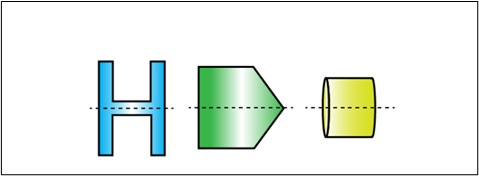Note

It is defined as the part of the horizontal line that is limited by the two points. Those two points are called the endpoints.

### Properties of Horizontal Lines

Horizontal Line always the form of y = k, and k is the y-intercept of the line

 General Formula for the equation of an Equation Line is y = k where k is the y-intercept
 Example 1

Find the equation of horizontal line whose y-intercept is (0, 2).

Solution:

Given:

Y-intercept = (0, 2)

We know that,

Generally, the equation of the horizontal line is y = k

Here, “k” is the y-intercept. So the value of k = 2

Finally, the equation of the horizontal line is y = 2.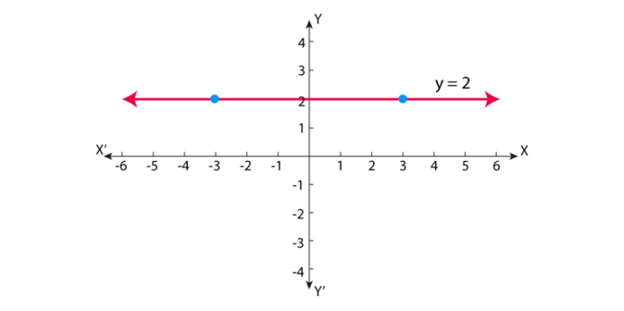## Vertical line test

The line parallel to the Y-axis is called a Vertical Line.

It is a straight line that goes from top to bottom and bottom to top.

The Vertical lines have no Slope. It goes parallelly along the Y-axis and hence the slope is not defined.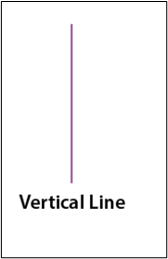The vertical line test is the method that is used to determine whether a given relation is a function or not. The approach is rather simple.

The vertical line test sustains the definition of a function, and finally, every x-value of a function must be paired to a single y-value. If we think of a vertical line as an infinite set of x-values, then intersecting the graph of a relation at exactly one point by a vertical line implies that a single x-value is only paired to a unique value of y.

In contrast, if the vertical line intersects the graph more than once this suggests that a single x-value is being associated with more than one value of y. This condition causes the relationship to be “disqualified” or not considered as a function.

If a vertical line passes the graph in all places at one point, then the relation is a function.

Let us look at some examples for vertical line test.

### Properties of Vertical Lines

• The equation of a vertical line in the graph, that is parallel to the y-axis is x = a.
• The slope of a vertical line is infinity or undefined as it has no y-intercept and the denominator in the slope formula is zero.
• To check whether the relation is a function, and we use a vertical line. It will be a function if all vertical lines intersect it minimum once.

This is also called a Vertical Line Test. A graph would be considered as a function if it has only one output y for each input x. Therefore, a vertical line cannot be a function.

 Example

Plot the graph for the following: (x = 3)?

Solution:

x=3 is a vertical line that passes through the x-axis, at a distance of 3 units on the right side of the origin and is parallel to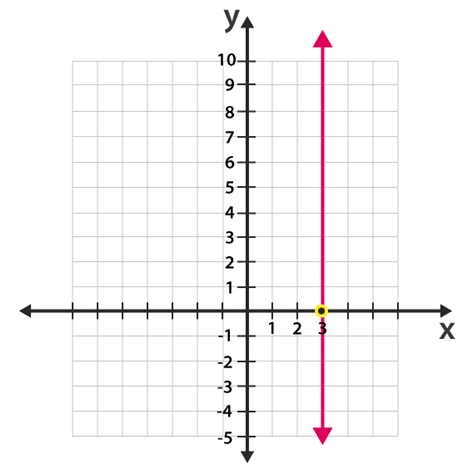## Horizontal Line Test, Vertical Line Test, One to One Function Test

If there are no different points in a graph and they have the same first coordinate, this means that vertical lines cross the graph at most once. This is known as the vertical line test. And the Graphs that pass the vertical line test are graphs of functions.

If no different points in a graph have the same second coordinate, that means that horizontal lines cross the graph at once. This is known as the horizontal line test.

Functions of the graphs that pass the horizontal line test are called one-to-one.

Graphs pass both the vertical line and horizontal line tests are known as one-to-one functions. These are exactly those functions where

Inverse relation is also known as a function and One-to-one functions have an inverse.

Let us explores some example graphs to chec if it is a function or not a function.

### Function Not One to One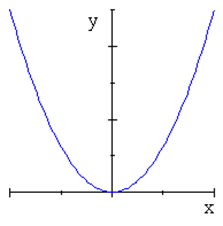### Not A Function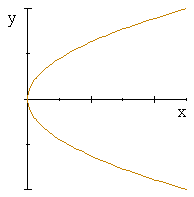### One-to-one function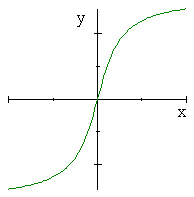### One-to-one function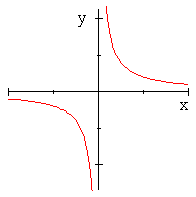## Summary

Basically, we have two types of line tests, Horizontal line test, and Vertical line test. And we have discussed all things related to them.

The vertical line test for functions is used to determine whether a given relation is a function or not. Horizontal line test is used to determine if a function is one to one and also to find if function is invertible with the inverse also being a function. And the line parallel to the x-axis is called a horizontal line.

Written by Neha Tyagi

## Vertical lines go which direction?

Vertical line goes from up to down or down to up.

## Horizontal lines go which direction?

Horizontal line goes from left to Right.

## Explain what the vertical line test is and how it is used.

The vertical line test for functions is used to find out if a relation is a function.

A vertical line is drawn for every value in the domain of relation and if that line only passes the relation once then the relation is a function.

## Does a vertical line pass the vertical line test?

No, a vertical line does not pass the vertical line test.

## Does a linear graph pass the vertical line test?

Yes a linear graph does pass the vertical line test.

## Explain why the vertical line test is used to determine if a graph represents a function.

If there are no different points in a graph and they have the same first coordinate, this means that vertical lines cross the graph at most once. This is known as the vertical line test. And the Graphs that pass the vertical line test are graphs of functions.

## Horizontal line test inverse

The horizontal line test is the simplest method using which we can determine whether a given function has an inverse, but more importantly to find out if the inverse is also a function.

GIVE YOUR CHILD THE CUEMATH EDGE
Access Personalised Math learning through interactive worksheets, gamified concepts and grade-wise courses
Learn More About Cuemath